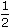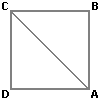# Aptitude - Area

### Exercise :: Area - General Questions

6.

The diagonal of the floor of a rectangular closet is 7feet. The shorter side of the closet is 4feet. What is the area of the closet in square feet?

A.
 5 1 4
B.
 13 1 2
C. 27
D. 37

Explanation:

Other side =152 -92 2 2
ft
=
 225 - 81 4 4
ft
=
 144 4
ft
= 6 ft.Area of closet = (6 x 4.5) sq. ft = 27 sq. ft.

7.

A towel, when bleached, was found to have lost 20% of its length and 10% of its breadth. The percentage of decrease in area is:

 A. 10% B. 10.08% C. 20% D. 28%

Explanation:

Let original length = x and original breadth = y.

Decrease in area
 = xy -80 x x 90 y100 100
 =xy - 18 xy25
 = 7 xy. 25Decrease % =7 xy x 1 x 100% = 28%. 25 xy

8.

A man walked diagonally across a square lot. Approximately, what was the percent saved by not walking along the edges?

 A. 20 B. 24 C. 30 D. 33

Explanation:

Let the side of the square(ABCD) be x metres.

Then, AB + BC = 2x metres.AC = 2x = (1.41x) m.

Saving on 2x metres = (0.59x) m.

 Saving % =0.59x x 100% = 30% (approx.) 2x

9.

The diagonal of a rectangle is 41 cm and its area is 20 sq. cm. The perimeter of the rectangle must be:

 A. 9 cm B. 18 cm C. 20 cm D. 41 cm

Explanation:

l2 + b2 = 41.

Also, lb = 20.

(l + b)2 = (l2 + b2) + 2lb = 41 + 40 = 81(l + b) = 9.Perimeter = 2(l + b) = 18 cm.

10.

What is the least number of squares tiles required to pave the floor of a room 15 m 17 cm long and 9 m 2 cm broad?

 A. 814 B. 820 C. 840 D. 844

Explanation:

Length of largest tile = H.C.F. of 1517 cm and 902 cm = 41 cm.

Area of each tile = (41 x 41) cm2.Required number of tiles =1517 x 902= 814. 41 x 41